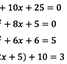Quadratics Practice 2016 Six questions, of progressive difficulty, each on 'complete the square then solve', 'factorise then solve', 'quadratic formula to solve'. PDF here.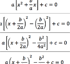Quadratic Formula Synthesis Complete the steps in the table to get from ax2+bx+c=0 to the quadratic formula. Or, cut out the steps and ask students to arrange them in the correct order. Answers on page 1, empty table on page 2, the peices in a random order on page 3. PDF hereCore 1 Quadratic Problem Solving Questions Five worded problems requiring knowledge of quadratic algebra to solve.Introduction to Solving Quadratics by Factorisation Examples (or use as questions) to lead classes through solving quadratics by factorising, from easier to much harder questions. Or use pdf version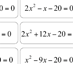Quadratics Factorising Vs Quadratic Formula A set of match up cards; quadratic equations and thier solutions, some solvable by factorising and others by the formula. Pupils decide which method to use.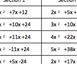Expanding / Factorising Quadratics where x coefficient is greater than 1 (expressions like 3x2+11x+10) A self-differentiable set of questions for students to practise their way through expanding and factorising quadratics, from straightforward to very difficult. Ask students to choose a path of adjacent questions leading from top-left (very easy) to bottom-right (fiendish). Answers included together with differing versions of the activity. Another set of factorising and expanding quadratics.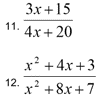Further Factorising Twenty questions to factorise the numerator and denominator quadratic expressions and then cancel. PDF HereQuadratic Discs Six questions to find everything out about the quadratic expressions.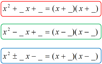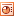Factorising Quadratic Types Prompt for learning where to put + & - symbols when factorising quadratics.Harder GCSE Algebra Question Match-up Cards A set of 26 harder algebra questions on simplifying, rearranging and solving. Pupils match up questions to answers and then use as support, or just for checking, to solve questions. PDF here. Here is set of 'rich questions' to go with the activity. Here are the questions sorted by type (and PDF here).Big Quadratic Formula Powerpoint slide of quadratic formula, very big for pupils to copy!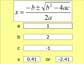Quadratic Formulae MS Excel program that makes quadratic formula easy - just input the a, b & c values and you're done! NB - Only use once you've practiced using the formula by hand first.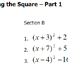Completing the Square Some questions that lead students to discover completing the square for themselves. Answers included. Practice at completing the square.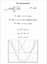The Discriminant Three examples of expressions with differing discriminants and thier affect on the graphs. Here is the Autograph file used.Quadratic Practice Three quadratic equations, of varying difficulty, to solve via each of the three methods: Factorising, Completing the Square & Quadratic Formula. Three versions of this: Easy, Medium or Hard. Practice at completing the square.Solving Quadratics by the 3 Methods Three sets of four questions: solving by factorising, solving by quadratic formula, solving by completing the square. In each set one question straightforward, one harder, one requiring rearrangment, one from another format. Answers included.Factorising Quadratics Lead In Ten quadratics to expand which lead to the concept of factorising with differing +/- symbols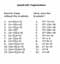Expanding Quadratics Twelve quadratic expansions to rewrite without the brackets. Then twelve more plus twelve to factorise.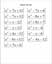Quadratic Matching Match up the factorised quadratic expressions with the expanded versions. I used this as a topic consolidation activity.Quadratic Expansions Ppt file that attempts to highlight similarities between long multiplication and expanding quadratics.Quadratics Cut them up then match up each expression in the expanded, factorised, completed square, graphical and the quadratic formula forms.